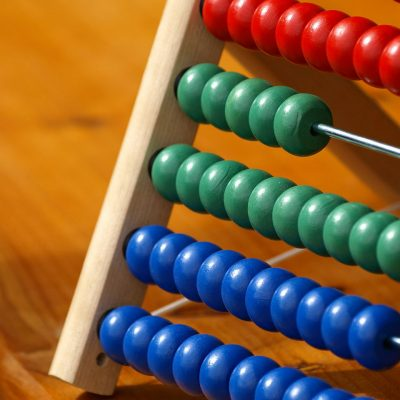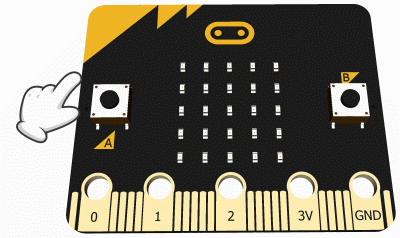### Simple multiplication### Assignment

Program the Micro Bit (as illustrated in the video assignment) in such a way that two randomly generated numbers (between 2 and 9) are multiplied (simple multiplication).### 1)Simple multiplication

Program the Micro Bit (as illustrated in the video assignment) in such a way that two randomly generated numbers (between 2 and 9) are multiplied (simple multiplication).#### Blockcode Info